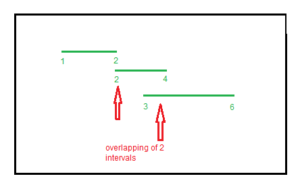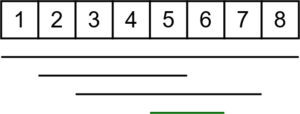# Maximum number of overlapping Intervals

Given different intervals, the task is to print the maximum number of overlap among these intervals at any time.

Examples:

Input: v = {{1, 2}, {2, 4}, {3, 6}}
Output: 2
The maximum overlapping is 2(between (1 2) and (2 4) or between (2 4) and (3 6))Input: v = {{1, 8}, {2, 5}, {5, 6}, {3, 7}}
Output: 4
The maximum overlapping is 4 (between (1, 8), (2, 5), (5, 6) and (3, 7))## Recommended: Please try your approach on {IDE} first, before moving on to the solution.

Approach:

• The idea is to store coordinates in a new vector of pair mapped with characters ‘x’ and ‘y’, to identify coordinates.
• Sort the vector.
• Traverse the vector, if an x coordinate is encountered it means a new range is added, so update count and if y coordinate is encountered that means a range is subtracted.
• Update the value of count for every new coordinate and take maximum.

## CPP

 `// C++ program that print maximum ` `// number of overlap ` `// among given ranges ` `#include ` `using` `namespace` `std; ` ` `  `// Function that print maximum ` `// overlap among ranges ` `void` `overlap(vector > v) ` `{ ` `    ``// variable to store the maximum ` `    ``// count ` `    ``int` `ans = 0; ` `    ``int` `count = 0; ` `    ``vector > data; ` ` `  `    ``// storing the x and y ` `    ``// coordinates in data vector ` `    ``for` `(``int` `i = 0; i < v.size(); i++) { ` ` `  `        ``// pushing the x coordinate ` `        ``data.push_back({ v[i].first, ``'x'` `}); ` ` `  `        ``// pushing the y coordinate ` `        ``data.push_back({ v[i].second, ``'y'` `}); ` `    ``} ` ` `  `    ``// sorting of ranges ` `    ``sort(data.begin(), data.end()); ` ` `  `    ``// Traverse the data vector to ` `    ``// count number of overlaps ` `    ``for` `(``int` `i = 0; i < data.size(); i++) { ` ` `  `        ``// if x occur it means a new range ` `        ``// is added so we increase count ` `        ``if` `(data[i].second == ``'x'``) ` `            ``count++; ` ` `  `        ``// if y occur it means a range ` `        ``// is ended so we decrease count ` `        ``if` `(data[i].second == ``'y'``) ` `            ``count--; ` ` `  `        ``// updating the value of ans ` `        ``// after every traversal ` `        ``ans = max(ans, count); ` `    ``} ` ` `  `    ``// printing the maximum value ` `    ``cout << ans << endl; ` `} ` ` `  `// Driver code ` `int` `main() ` `{ ` `    ``vector > v ` `        ``= { { 1, 2 }, { 2, 4 }, { 3, 6 } }; ` `    ``overlap(v); ` `    ``return` `0; ` `} `

## Python3

 `# Python3 program that prmaximum ` `# number of overlap ` `# among given ranges ` ` `  `# Function that prmaximum ` `# overlap among ranges ` `def` `overlap(v): ` ` `  `    ``# variable to store the maximum ` `    ``# count ` `    ``ans ``=` `0` `    ``count ``=` `0` `    ``data ``=` `[] ` ` `  `    ``# storing the x and y ` `    ``# coordinates in data vector ` `    ``for` `i ``in` `range``(``len``(v)): ` ` `  `        ``# pushing the x coordinate ` `        ``data.append([v[i][``0``], ``'x'``]) ` ` `  `        ``# pushing the y coordinate ` `        ``data.append([v[i][``1``], ``'y'``]) ` ` `  `    ``# sorting of ranges ` `    ``data ``=` `sorted``(data) ` ` `  `    ``# Traverse the data vector to ` `    ``# count number of overlaps ` `    ``for` `i ``in` `range``(``len``(data)): ` ` `  `        ``# if x occur it means a new range ` `        ``# is added so we increase count ` `        ``if` `(data[i][``1``] ``=``=` `'x'``): ` `            ``count ``+``=` `1` ` `  `        ``# if y occur it means a range ` `        ``# is ended so we decrease count ` `        ``if` `(data[i][``1``] ``=``=` `'y'``): ` `            ``count ``-``=` `1` ` `  `        ``# updating the value of ans ` `        ``# after every traversal ` `        ``ans ``=` `max``(ans, count) ` ` `  `    ``# printing the maximum value ` `    ``print``(ans) ` ` `  `# Driver code ` `v ``=` `[ [ ``1``, ``2` `], [ ``2``, ``4` `], [ ``3``, ``6` `] ] ` `overlap(v) ` ` `  `# This code is contributed by mohit kumar 29 `

Output:

```2
```

GeeksforGeeks has prepared a complete interview preparation course with premium videos, theory, practice problems, TA support and many more features. Please refer Placement 100 for details

My Personal Notes arrow_drop_upCheck out this Author's contributed articles.

If you like GeeksforGeeks and would like to contribute, you can also write an article using contribute.geeksforgeeks.org or mail your article to contribute@geeksforgeeks.org. See your article appearing on the GeeksforGeeks main page and help other Geeks.

Please Improve this article if you find anything incorrect by clicking on the "Improve Article" button below.

Improved By : mohit kumar 29

Article Tags :
Practice Tags :

1

Please write to us at contribute@geeksforgeeks.org to report any issue with the above content.